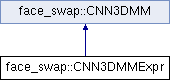Nirkin Face Swap  0.9.0 Face swap.
face_swap::CNN3DMMExpr Class Reference

This class provided face shape, texture, expression and pose estimations using Caffe with a convolutional neural network. More...

`#include <cnn_3dmm_expr.h>`

Inheritance diagram for face_swap::CNN3DMMExpr:## Public Member Functions

CNN3DMMExpr (const std::string &deploy_file, const std::string &caffe_model_file, const std::string &mean_file, const std::string &model_file, bool generic=false, bool with_expr=true, bool with_gpu=true, int gpu_device_id=0)
Creates an instance of CNN3DMMExpr. More...

~CNN3DMMExpr ()
Destructor.

void process (const cv::Mat &img, const std::vector< cv::Point > &landmarks, cv::Mat &shape_coefficients, cv::Mat &tex_coefficients, cv::Mat &expr_coefficients, cv::Mat &vecR, cv::Mat &vecT, cv::Mat &K)
Estimate face pose and shape, texture, expression coefficients from image. More...Public Member Functions inherited from face_swap::CNN3DMM
CNN3DMM (const std::string &deploy_file, const std::string &caffe_model_file, const std::string &mean_file, bool init_cnn=true, bool with_gpu=true, int gpu_device_id=0)
Creates an instance of CNN3DMM. More...

~CNN3DMM ()
Destructor.

void process (const cv::Mat &img, cv::Mat &shape_coefficients, cv::Mat &tex_coefficients)
Estimate face shape and texture from image. More...Protected Attributes inherited from face_swap::CNN3DMM
std::shared_ptr< caffe::Net< float > > m_net

int m_num_channels

cv::Size m_input_size

cv::Mat m_mean

bool m_with_gpu

## Detailed Description

This class provided face shape, texture, expression and pose estimations using Caffe with a convolutional neural network.

The CNN estimates shape and texture coefficients for a PCA model that is based on Basel's 3D Morphable Model. The pose and expression are then estimated using epnp optimization. This is an implementation of the following papers:

1. Regressing Robust and Discriminative 3D Morphable Models with a very Deep Neural Network, Anh Tuan Tran, Tal Hassner, Iacopo Masi and Gerard Medioni.
2. A 3D Face Model for Pose and Illumination Invariant Face Recognition, P. Paysan and R. Knothe and B. Amberg and S. Romdhani and T. Vetter.

## Constructor & Destructor Documentation

 face_swap::CNN3DMMExpr::CNN3DMMExpr ( const std::string & deploy_file, const std::string & caffe_model_file, const std::string & mean_file, const std::string & model_file, bool generic = `false`, bool with_expr = `true`, bool with_gpu = `true`, int gpu_device_id = `0` )

Creates an instance of CNN3DMMExpr.

Parameters
 deploy_file Path to 3DMM regression CNN deploy file (.prototxt). caffe_model_file Path to 3DMM regression CNN model file (.caffemodel). mean_file Path to 3DMM regression CNN mean file (.binaryproto). model_file Path to 3DMM file (.dat). generic Use generic model without shape regression. with_expr Toggle fitting face expressions. with_gpu Toggle GPU execution. gpu_device_id Set the GPU's device id.

## Member Function Documentation

 void face_swap::CNN3DMMExpr::process ( const cv::Mat & img, const std::vector< cv::Point > & landmarks, cv::Mat & shape_coefficients, cv::Mat & tex_coefficients, cv::Mat & expr_coefficients, cv::Mat & vecR, cv::Mat & vecT, cv::Mat & K )

Estimate face pose and shape, texture, expression coefficients from image.

Parameters
 [in] img The image to process. [in] landmarks The face landmarks detected on the specified image. [out] shape_coefficients PCA shape coefficients. [out] tex_coefficients PCA texture coefficients. [out] expr_coefficients PCA expression coefficients. [out] vecR Face's rotation vector [Euler angles]. [out] vecT Face's translation vector. [out] K Camera intrinsic parameters.

The documentation for this class was generated from the following file: